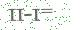# SUMIF函数还能这么用。

 后台-系统设置-扩展变量-手机广告位-内容正文顶部

#### SUMIF函数还能这么用。

EH看见星光 Excel星球
Excel星球AhaExcelNO.816-SUMIF

微博：EXCELers / 知识星球：Excel

HI，大家好，我是星光。= SUMIF(A:A,"不好说",D:D) + SUMIF(A:A,"说不好",D:D)

=SUM(SUMIF(A:A, {"不好说","说不好"} ,D:D))

……

{"不好说","说不好"} 是SUMIF函数的第2参数，表示查询条件。{}表示常量数组，里面包含两个元素： "不好说" "说不好" 。SUMIF分别统计这两者的奖金总额，返回一个内存数组：

`{198,176}`↑ 一帖带你全面了解函数数组那些事

……

❶   SUMIFS函数：

``` =SUM(SUMIFS(D:D, A :A,{ "不好说" , "说不好" })) ```

❷  SUM函数：

``` =SUM((A2:A19={ "不好说" , "说不好" })*D2:D19) ```

SUMPRODUCT函数（1）：

`=SUMPRODUCT((A2:A19={"不好说","说不好"})*D2:D19)`

❹  SUMPRODUCT函数（2）

``` =SUMPRODUCT((ISNUMBER(FIND(A2:A19, "不好说,说不好" ))*D2:D19)) ```

FILTER函数：

``` =SUM( FILTER (D2:D19,ISNUMBER(FIND(A2:A19, "不好说,说不好" )))) ```

❻  MMLUT函数：

``` =SUM(MMULT(TRANSPOSE(D2:D19), 1 *(A2:A19={ "不好说" , "说不好" }))) ```

……

01：SUMIF(S)用法技巧全整理
02：一帖带你学习SUMPRODUCT
03：函数数组那些事儿
04：超级函数FILTER

https://pan.baidu.com/s/1WMaYEzazmDphtOzI7QMLkQ后台-系统设置-扩展变量-手机广告位-内容正文底部
 留言与评论（共有 0 条评论）

 验证码：